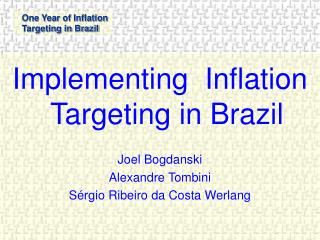# Implementing Inflation Targeting in Brazil Joel Bogdanski Alexandre Tombini Sérgio Ribeiro da Costa Werlang - PowerPoint PPT PresentationDownload PresentationImplementing Inflation Targeting in Brazil Joel Bogdanski Alexandre Tombini Sérgio Ribeiro da Costa Werlang

Download Presentation## Implementing Inflation Targeting in Brazil Joel Bogdanski Alexandre Tombini Sérgio Ribeiro da Costa Werlang

- - - - - - - - - - - - - - - - - - - - - - - - - - - E N D - - - - - - - - - - - - - - - - - - - - - - - - - - -
##### Presentation Transcript

1. Implementing Inflation Targeting in Brazil Joel Bogdanski Alexandre Tombini Sérgio Ribeiro da Costa Werlang

2. Macroeconomic Models • Instrumental for managing monetary policy under IT • Powerful tool for communicating monetary policy (inflation fan charts)

3. Challenges for Macro Modeling • Exchange-rate passthrough • Endogeneization of exchange-rate movements • Forward vs. Background-looking Phillips curve • Role of inflation expectations • Role of prices set by the public sector

4. Building Blocks • Demand (IS Curve) • Supply (Phillips Curve) • exchange-rate passthrough • forward x backward looking • inflation expectation • Exchange-rate (UIP) • endogenous x exogenous risk premium • Interest rate rules • Taylor type rules • predetermined path • optimal rules

5. Demand Side (IS curve) • Non Fiscal IS • Fiscal IS where: h log of output gap. r log of (one plus) real interest rate Pr  log of (one plus) total primary deficit /GDP h,hf white noise.

6. Supply Side (Phillips Curve) • Backward-looking • Forward-looking • Combined where:  log of one plus inflation. h log of output gap. pF log of foreign producer price index e log of exchange rate. Et(.)  Expectation on time t. b, f, n white noise.

7. Modeling the passthrough where: pF log of foreign producer price index. e log of exchange-rate. E exchange-rate.

8. Treatment of inflation expectations • Forward-looking Phillips curve • Alternatives • Institutional approach

9. Treatment of inflation expectations • Alternatives • Model Consistent (recursive solution)* where ab means that b is in a neighborhood of a. * - The convergence is usually achieved in less than 20 iterations.

10. Exchange-rate determination • Exchange rate follows a UIP: where: e log of exchange rate iF log of foreign interest rate x log of risk premium  residual including the expectation variations assumed white noise

11. Exchange-rate determination • Modeling the risk premium • exogenous path • endogenous determination • depends on PSBR/GDP ratio (primary) and other risk premium determinants. where: X  risk premium (SOT) in basis points PR PSBR/GDP ratio (primary) Zj other risk premium determinants

12. Interest rate rules • Taylor type rules where:  log of inflation * log of inflation target h log of output gap i log of interest rate   degree of interest rate smoothing ( = 1, conventional Taylor rule) ’s  arbitrarily set or obtained through an optimization procedure • Predetermined path • fixed nominal rate (fan chart) • budget trajectory

13. Interest rate rules • Optimal rules • Non-stochastic simulation: find an interest rate path that minimizes the following loss-function. • Stochastic simulation: find an interest rate path that minimizes the following loss function. This simulation is more computer demanding than the non-stochastic one.

14. Forecasting • Scenarios • Model specification • Copom defines which relations are relevant for the monetary policy decision. • Exogenous variables • The most likely path for the exogenous variables are set by the Copom after interacting with the staff. • Shocks • The timing, magnitude, variance and skewness are set by the Copom after interacting with the staff.

15. Forecasting • Fan Chart • Measure of central tendency • median: the model estimate the mean, median is obtained using the variance and skewness of a two-piece normal distribution. • Shocks stylization • The magnitudes are obtained from out of model estimation. The assessment of variance and skewness are subjective. • Variance • It is calculated using the historical forecast error as benchmark. However, it can be adjusted by subjective assessment.